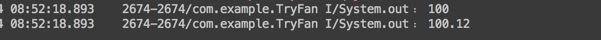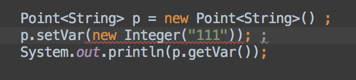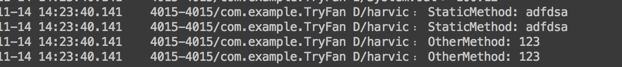# 夯实JAVA基本之一 —— 泛型详解(1):基本使用

## 一、引入

### 1、泛型是什么

ArrayList<String> strList = new ArrayList<String>();
ArrayList<Integer> intList = new ArrayList<Integer>();
ArrayList<Double> doubleList = new ArrayList<Double>();

### 2、没有泛型会怎样

//设置Integer类型的点坐标
class IntegerPoint{
private Integer x ;       // 表示X坐标
private Integer y ;       // 表示Y坐标
public void setX(Integer x){
this.x = x ;
}
public void setY(Integer y){
this.y = y ;
}
public Integer getX(){
return this.x ;
}
public Integer getY(){
return this.y ;
}
}
//设置Float类型的点坐标
class FloatPoint{
private Float x ;       // 表示X坐标
private Float y ;       // 表示Y坐标
public void setX(Float x){
this.x = x ;
}
public void setY(Float y){
this.y = y ;
}
public Float getX(){
return this.x ;
}
public Float getY(){
return this.y ;
}
}

class ObjectPoint{
private Object x ;
private Object y ;
public void setX(Object x){
this.x = x ;
}
public void setY(Object y){
this.y = y ;
}
public Object getX(){
return this.x ;
}
public Object getY(){
return this.y ;
}
}

ObjectPoint integerPoint = new ObjectPoint();
integerPoint.setX(new Integer(100));
Integer integerX=(Integer)integerPoint.getX();

integerPoint.setX(new Integer(100));

Integer integerX=(Integer)integerPoint.getX();

ObjectPoint floatPoint = new ObjectPoint();
floatPoint.setX(new Float(100.12f));
Float floatX = (Float)floatPoint.getX();

ObjectPoint floatPoint = new ObjectPoint();
floatPoint.setX(new Float(100.12f));
String floatX = (String)floatPoint.getX();

String floatX = (String)floatPoint.getX();

## 二、各种泛型定义及使用

### 1、泛型类定义及使用

//定义
class Point<T>{// 此处可以随便写标识符号
private T x ;
private T y ;
public void setX(T x){//作为参数
this.x = x ;
}
public void setY(T y){
this.y = y ;
}
public T getX(){//作为返回值
return this.x ;
}
public T getY(){
return this.y ;
}
};
//IntegerPoint使用
Point<Integer> p = new Point<Integer>() ;
p.setX(new Integer(100)) ;
System.out.println(p.getX());

//FloatPoint使用
Point<Float> p = new Point<Float>() ;
p.setX(new Float(100.12f)) ;
System.out.println(p.getX());（1）、定义泛型：Point<T>

（2）类中使用泛型

//定义变量
private T x ;
//作为返回值
public T getX(){
return x ;
}
//作为参数
public void setX(T x){
this.x = x ;
} 

（3）使用泛型类

//IntegerPoint使用
Point<Integer> p = new Point<Integer>() ;
p.setX(new Integer(100)) ;
System.out.println(p.getX());

//FloatPoint使用
Point<Float> p = new Point<Float>() ;
p.setX(new Float(100.12f)) ;
System.out.println(p.getX());  

Point<String> p = new Point<String>() ; 

//IntegerPoint使用
Point<Integer> p = new Point<Integer>() ;
//FloatPoint使用
Point<Float> p = new Point<Float>() ; 

public class ArrayList<E>{
…………
}

(4）使用泛型实现的优势

（1）、不用强制转换

//使用Object作为返回值，要强制转换成指定类型
Float floatX = (Float)floatPoint.getX();
//使用泛型时，不用强制转换，直接出来就是String
System.out.println(p.getVar()); 

(2)、在settVar()时如果传入类型不对，编译时会报错### 2、多泛型变量定义及字母规范

（1）、多泛型变量定义

class MorePoint<T,U>{
}

class MorePoint<T,U,A,B,C>{
}

class MorePoint<T,U> {
private T x;
private T y;

private U name;

public void setX(T x) {
this.x = x;
}
public T getX() {
return this.x;
}
…………
public void setName(U name){
this.name = name;
}

public U getName() {
return this.name;
}
}
//使用
MorePoint<Integer,String> morePoint = new MorePoint<Integer, String>();
morePoint.setName("harvic");
Log.d(TAG, "morPont.getName:" + morePoint.getName());

（2）、字母规范

class Point<T>{
…………
}

•  E — Element，常用在java Collection里，如：List<E>,Iterator<E>,Set<E>
•  K,V — Key，Value，代表Map的键值对
•  N — Number，数字
•  T — Type，类型，如String，Integer等等

### 3、泛型接口定义及使用

interface Info<T>{        // 在接口上定义泛型
public T getVar() ; // 定义抽象方法，抽象方法的返回值就是泛型类型
public void setVar(T x);
}  

（1）、使用方法一：非泛型类

class InfoImpl implements Info<String>{	// 定义泛型接口的子类
private String var ;				// 定义属性
public InfoImpl(String var){		// 通过构造方法设置属性内容
this.setVar(var) ;
}
@Override
public void setVar(String var){
this.var = var ;
}
@Override
public String getVar(){
return this.var ;
}
}

public class GenericsDemo24{
public  void main(String arsg[]){
InfoImpl i = new InfoImpl("harvic");
System.out.println(i.getVar()) ;
}
};

class InfoImpl implements Info<String>{
…………
}

public class GenericsDemo24{
public  void main(String arsg[]){
InfoImpl i = new InfoImpl("harvic");
System.out.println(i.getVar()) ;
}
};

（2）、使用方法二：泛型类

interface Info<T>{		// 在接口上定义泛型
public T getVar() ;	// 定义抽象方法，抽象方法的返回值就是泛型类型
public void setVar(T var);
}
class InfoImpl<T> implements Info<T>{	// 定义泛型接口的子类
private T var ;				// 定义属性
public InfoImpl(T var){		// 通过构造方法设置属性内容
this.setVar(var) ;
}
public void setVar(T var){
this.var = var ;
}
public T getVar(){
return this.var ;
}
}
public class GenericsDemo24{
public static void main(String arsg[]){
InfoImpl<String> i = new InfoImpl<String>("harvic");
System.out.println(i.getVar()) ;
}
};

class InfoImpl<T> implements Info<T>{	// 定义泛型接口的子类
private T var ;				// 定义属性
public InfoImpl(T var){		// 通过构造方法设置属性内容
this.setVar(var) ;
}
public void setVar(T var){
this.var = var ;
}
public T getVar(){
return this.var ;
}
}

public class GenericsDemo24{
public static void main(String arsg[]){
Info<String> i = new InfoImpl<String>("harvic");
System.out.println(i.getVar()) ;
}
};

class InfoImpl<T,K,U> implements Info<U>{	// 定义泛型接口的子类
private U var ;
private T x;
private K y;
public InfoImpl(U var){		// 通过构造方法设置属性内容
this.setVar(var) ;
}
public void setVar(U var){
this.var = var ;
}
public U getVar(){
return this.var ;
}
}

public class GenericsDemo24{
public  void main(String arsg[]){
InfoImpl<Integer,Double,String> i = new InfoImpl<Integer,Double,String>("harvic");
System.out.println(i.getVar()) ;
}
}

### 4、泛型函数定义及使用

public class StaticFans {
//静态函数
public static  <T> void StaticMethod(T a){
Log.d("harvic","StaticMethod: "+a.toString());
}
//普通函数
public  <T> void OtherMethod(T a){
Log.d("harvic","OtherMethod: "+a.toString());
}
}

//静态方法
StaticFans.StaticMethod("adfdsa");//使用方法一
StaticFans.<String>StaticMethod("adfdsa");//使用方法二

//常规方法
StaticFans staticFans = new StaticFans();
staticFans.OtherMethod(new Integer(123));//使用方法一
staticFans.<Integer>OtherMethod(new Integer(123));//使用方法二StaticFans.StaticMethod("adfdsa");//使用方法一
StaticFans.<String>StaticMethod("adfdsa");//使用方法二

StaticFans staticFans = new StaticFans();
staticFans.OtherMethod(new Integer(123));//使用方法一
staticFans.<Integer>OtherMethod(new Integer(123));//使用方法二

public static <T> List<T> parseArray(String response,Class<T> object){
List<T> modelList = JSON.parseArray(response, object);
return modelList;
}

### 5、其它用法:Class<T>类传递及泛型数组

（1）、使用Class<T>传递泛型类Class对象

public static List<SuccessModel> parseArray(String response){
List<SuccessModel> modelList = JSON.parseArray(response, SuccessModel.class);
return modelList;
}

public class SuccessModel {
private boolean success;

public boolean isSuccess() {
return success;
}

public void setSuccess(boolean success) {
this.success = success;
}
} 

public static List<SuccessModel> parseArray(String response){
List<SuccessModel> modelList = JSON.parseArray(response, SuccessModel.class);
return modelList;
}

public static <T> List<T> parseArray(String response,Class<T> object){
List<T> modelList = JSON.parseArray(response, object);
return modelList;
}

public final class Class<T> implements Serializable {
…………
}

（2）、定义泛型数组

//定义
public static <T> T[] fun1(T...arg){  // 接收可变参数
return arg ;            // 返回泛型数组
}
//使用
public static void main(String args[]){
Integer i[] = fun1(1,2,3,4,5,6) ;
Integer[] result = fun1(i) ;
}  

public static <T> T[] fun1(T...arg){  // 接收可变参数
return arg ;            // 返回泛型数组
}  

## 如果你喜欢我的文章，你可能更喜欢我的公众号06-2111-243448
09-18
04-01
08-03129
06-222万+
12-3043万+
07-083164
08-1781
03-303万+
10-01627
03-274011
12-191074
09-25190
06-211431
06-03211
10-24336
12-10133
©️2020 CSDN 皮肤主题: 大白 设计师:CSDN官方博客